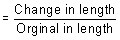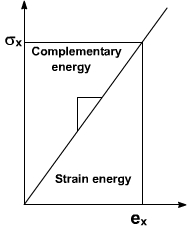# Strain Energy

### Strain Energy

Strain Energy of the member is defined as the internal work done in defoming the body by the action of externally applied forces. This energy in elastic bodies is known as elastic strain energy :

Let as consider an infinitesimal element of dimensions as shown in Fig .1. Let the element be subjected to normal stress σx.

The forces acting on the face of this element is σx. dy. dz

where dydz = Area of the element due to the application of forces, the element deforms to an amount = ∈x dx

∈x = strain in the material in x – directionAssuming the element material to be as linearly elastic the stress is directly proportional to strain as shown in Fig . 2.### Feedback is important to us.#### Shiva

I love to write technical articles.

error: Content is protected !!